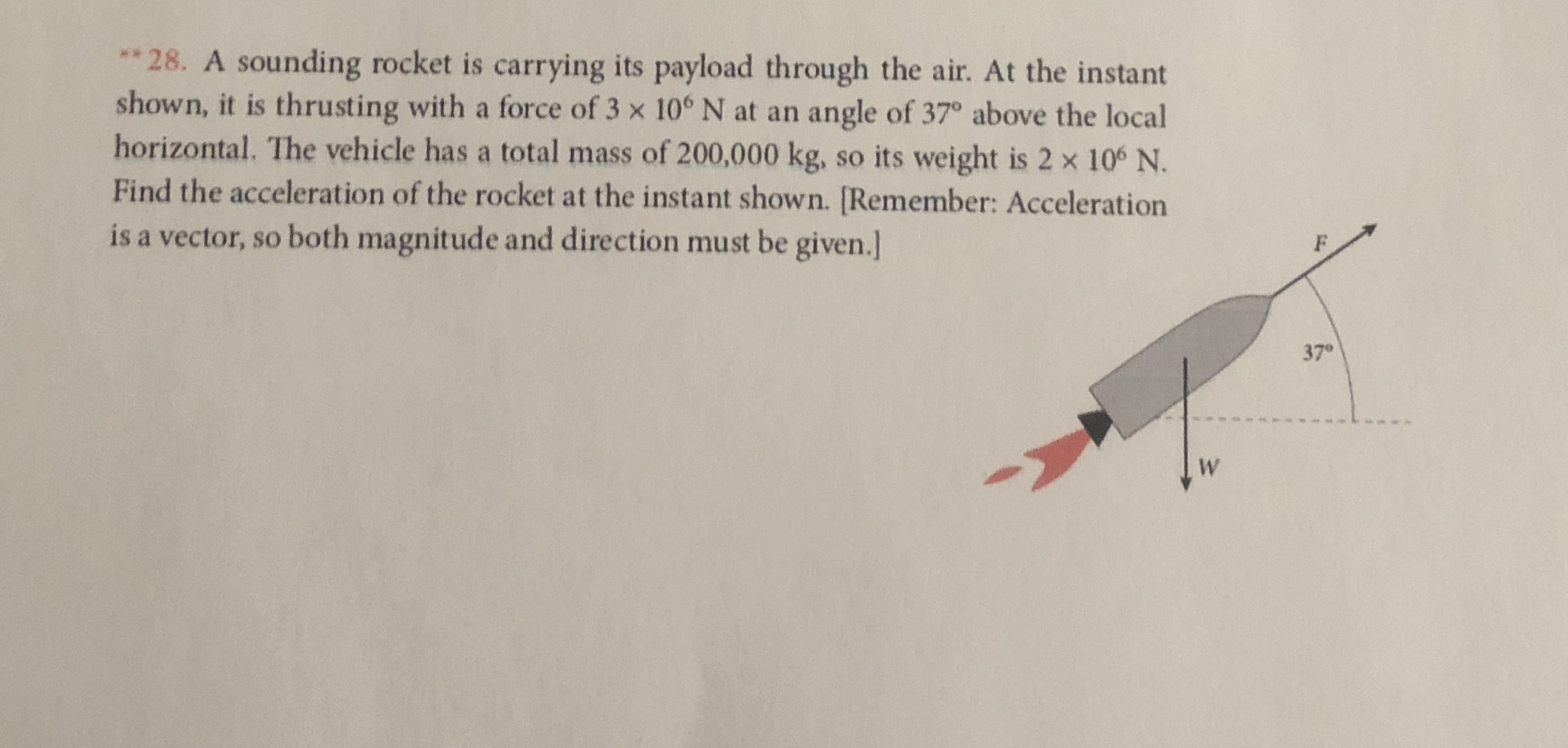# ** 28. A sounding rocket is carrying its payload through the air. At the instantshown, it is thrusting with a force of 3 x 10° N at an angle of 37° above the localhorizontal. The vehicle has a total mass of 200,000 kg,Find the acceleration of the rocket at the instant shown. [Remember: Accelerationso its weight is 2 x 106 N.is a vector, so both magnitude and direction must be given.]37°W

Question
9 viewshelp_outlineImage Transcriptionclose** 28. A sounding rocket is carrying its payload through the air. At the instant shown, it is thrusting with a force of 3 x 10° N at an angle of 37° above the local horizontal. The vehicle has a total mass of 200,000 kg, Find the acceleration of the rocket at the instant shown. [Remember: Acceleration so its weight is 2 x 106 N. is a vector, so both magnitude and direction must be given.] 37° W fullscreen
check_circle

Step 1

Horizontal component of acceleration is,

Step 2

Substituting the values,

Step 3

Gravitational acceleration is acting vertically downwards. Note that the acceleration component in vertically upward direction is consi...

### Want to see the full answer?

See Solution

#### Want to see this answer and more?

Solutions are written by subject experts who are available 24/7. Questions are typically answered within 1 hour.*

See Solution
*Response times may vary by subject and question.
Tagged in

### Physics